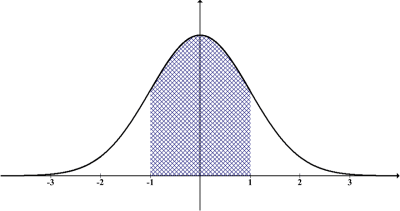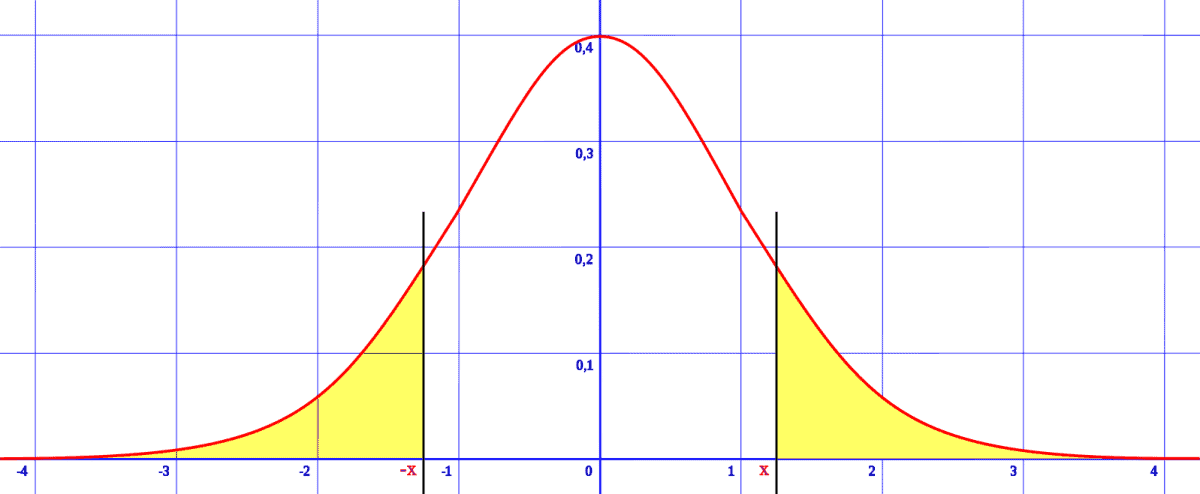# Can A Normal Distribution Be Negative? (3 Things To Know)

Normal distributions are quite common, and they show up everywhere in statistics and in nature.  We are familiar with distributions with positive values (such as height or weight), but what about negative values?

So, can a normal distribution be negative?  A normal distribution can have negative values for some or all of its data points.  A normal distribution can also have a negative mean.  However, the standard deviation of a normal distribution is always positive – it is never negative or zero.

Of course, for a normal distribution to have a negative mean, there would need to be some data points with negative values.

In this article, we’ll talk about normal distributions and when they can be negative.  We’ll also take a look at some examples to help make things clear.

Let’s get going.

## Can A Normal Distribution Be Negative?

A normal distribution can have negative values for some or all of its data points.  It is also possible for the mean (expected value) of a normal distribution to be negative.A normal distribution can have negative values, and in some cases it can have a negative mean. All of the values to the left of the mean (zero) on a standard normal distribution are negative.

However, this would require at least some of the data points to have negative values.  After all, a distribution with all positive values cannot have a negative mean.

It is important to remember that the standard deviation of a normal distribution is always positive.  It cannot be negative or zero.

(If the standard deviation were zero, we would have a uniform distribution with constant value, not a normal distribution).

### Can A Normal Distribution Have Negative Values?

A normal distribution can have negative values for some or all of its data points.

Here are some examples of normal distributions with negative values.

#### Example 1: Negative Of Heights

Let H be a normal distribution of heights.  Since heights are always positive, we know that every data point in this distribution is positive.

Let Y = -H be the negative of heights.  Since all heights are positive, taking the negative will always give us negative values.

So, the negative of heights has all negative values.

#### Example 2: Stock Market Returns

If we assume stock rates of return are normally distributed, they could have positive return, negative return, or zero return in a given year.

For example:

• A stock that has a value of \$100 on January 1, 2020 and a value of \$115 on December 31, 2020 has a 15% (positive) return for the year 2020.
• A stock that has a value of \$100 on January 1, 2020 and a value of \$100 on December 31, 2020 has a 0% (zero) return for the year 2020.
• A stock that has a value of \$100 on January 1, 2020 and a value of \$75 on December 31, 2020 has a -25% (negative) return for the year 2020.

Even if the mean return is positive, there can still be a year when a stock has a negative rate of return.

#### Example 3: Standard Normal Distribution

The standard normal distribution has a mean of 0 and a standard deviation of 1.  This makes it easy to work with and allows us to work with a standard normal table.Converting our data to a standard normal distribution makes it easy to find probabilities by using the standard normal table.

Remember that any normal distribution is symmetric, and the mean and median are the same.  Since the mean of a standard normal distribution is zero, then the median is zero as well.

The median is the “middle” of a distribution: 50% of data points are greater than the median and 50% of data points are less than the median.

So, 50% of all data points in a standard normal distribution are less than the median of zero (that is, they are negative).

Recall that the standard normal distribution comes from “standardizing” all data points in a normal distribution by subtracting the mean and dividing by the standard deviation.

If the original distribution (denoted by the variable X) had a mean of M and a standard deviation of S, then the new variable Z = (X – M) / S has a standard normal distribution with a mean of 0 and a standard deviation of 1.

### Can The Mean Of A Normal Distribution Be Negative?

The mean of a normal distribution can be negative.  This is guaranteed to happen if every data point has a negative value, and it can happen when only some of the data points are negative.

Note that if the mean of a normal distribution is negative, then more than 50% of the data points in the distribution are negative.

Negative values for some data points are required to have a negative mean.  If every data point were positive (such as for height or weight), then the mean would be positive.

#### Example 1: Negative Of Weights

Let W be a normal distribution of weights.  Since weights are always positive, we know that every data point in this distribution is positive.

Let Y = -W be the negative of weights.  Since all weights are positive, taking the negative will always give us negative values.

So, the negative of weights has all negative values.  When we find the mean of these negative values, the outcome is guaranteed to be negative.

Thus, the negative of weights is a normal distribution with negative mean.  (Note: it also has the same standard deviation as the original distribution of positive weights).

#### Example 2: Weight Change

Let X be a normal distribution of weight change in a year by a group of people.  A positive value would mean weight gain, a negative value would mean weight loss, and a zero value would mean no change in weight during the year.

It is possible that the average person lost weight.  For example, the mean weight change could be -2 pounds, and the standard deviation could be 4 pounds.

The mean of this distribution is negative, and more than half of the data points have a negative value.

In fact, we can find out the percentage of weight changes that are negative by converting to the standard normal (subtract mean and divide by standard deviation):

• The desired value is 0 (since less than 0 is negative).
• The mean is -2.
• The standard deviation is 4.

This gives us a z-score of Z = (0 – (-2)) / 4 = ½ = 0.5.  The probability of Z <= 0.5 is 0.6915, or 69.15%.

So, 69.15% of people lost weight (or stayed the same weight).

### Can Standard Deviation Be Negative?

Standard deviation for a normal distribution cannot be negative or zero.  The standard deviation is always positive for a normal distribution.

A standard deviation of zero means that all data points are the same.  In that case, you would have a uniform distribution, with one constant value.

Remember that standard deviation comes from taking a positive square root (and it involves adding up squared values, which are always positive or zero).  This means that standard deviation will be positive for a normal distribution.

### Can Mean Minus Standard Deviation Be Negative?

The mean minus the standard deviation can be negative.  This implies that either:

• 1. The mean is negative (standard deviation is always positive, so a negative mean minus a positive standard deviation will always yield a negative result).
• 2. The mean positive or zero and it is less than the standard deviation (subtracting a larger positive number from a smaller positive number will always yield a negative result).

In either case, this scenario will imply that at least some of the data points in the distribution will have negative values.

Keep in mind that this situation only makes sense with a distribution where the values can actually be negative.

Remember that heights are always positive, so if the standard deviation is larger than the mean, then you may want to check your calculations or use another distribution to model the data.

The rectified Gaussian (normal) distribution tries to make sense of this by replacing negative values with zero.

Here are some examples of distributions where the mean minus the standard deviation is negative.

#### Example 1: Negative Mean

Consider the distribution of weight changes we mentioned earlier.  The mean was -2 pounds and standard deviation was 4 pounds.

Then mean minus standard deviation is -2 – 4 = -6, which is negative.

#### Example 2: Mean Less Than Standard Deviation

Consider a normal distribution with a mean of 20 and a standard deviation of 30.  Then the mean minus the standard deviation is 20 – 30 = -10, which is negative.

## Conclusion

Now you know about when normal distributions and their parameters can be negative.

You can learn about how to work with normal distributions in Excel here.

Speaking of negative numbers, you might also want to check out my article on absolute value and negative numbers.